A Stochastic Model in Discrete States and Discrete Time for the Control of Leprosy Disease

Usman Yusuf ABUBAKAR

Department of Mathematics and Computer Science, Federal University of Technology, Minna, Nigeria

abbkruy@yahoo.com

Abstract

This paper presents the use of Semi-Markov model in discrete state and discrete time to study the condition of leprosy patients. Leprosy is considered to be controlled if is possible to maximize recovery and consequently minimize relapse and death. The model takes into account the concept of effectiveness of the treatment. The result shows that it is possible to attain a zero probabilities for a relapse and death from leprosy when the treatment is very effective. The model could be used to predict the cases of leprosy disease. The predictions could be used for planning resources for the control of leprosy.

Keywords

Stochastic; Semi-Markov; Interval; Transition; Probability; Leprosy, Control

Introduction

Policy makers and administrators in the health industry take into account quantitative predictions from stochastic models on decision-making policies. Leprosy is an infectious disease of the skin and nerves. It is caused by a mycobacterium leprae, often resulting into the deformity of the body peripherals like the fingers and toes. It can be treated and cured.

Several mathematical studies of leprosy have been reported including  that have studied the prevalence rate and the incidence of new cases. A study on the Leprosy rates in villages of different distance apart has been presented in . Semi-Markov models have been used to study relapses from leprosy after treatment with Dapsone and MDT regimen respectively, . In this paper, we apply the principles of semi-markov process in discrete states and time to study cases of recovery and death in addition to relapses from leprosy.

The model

Consider stochastic process íXn, n = 0, 1, 2, …ý that takes on a finite or countable number of possible values such that: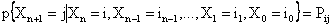(1)

for all states i0, i1,…,in-1,i,j and all n≥0. Such a stochastic process is known as a Markov chain . Since probabilities are nonnegative and since the process must make a transition into some state, we have that.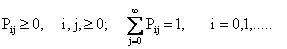Let us consider a process whose successive state occupancies are governed by the transition probabilities of a Markov chains in (1), but whose stay in any state is described by a random variable that depends on the state to which the next transition is made such a process is referred to as a semi-Markov process .

Whenever the patient enters state ‘i’ he remains there for a time Tij in state i before making a transition to state ‘j’. Tij  is called the ‘holding time’ in state i. The holding times are positive integer valued random variables each governed by a probability distribution function fij ( ) called the holding time distribution function for a transition from state i to state j.. Thus P(Tij = m) = fij (m).  m = 1, 2, 3, . . . i, j =1, 2, 3, 4.

The states transition probabilities and the holding time in the states before the transition is made describes a semi-Markov process. Thus, we have to specify holding time distribution functions in addition to the transition probabilities. For a fixed value of i, Tij is the same for each value of j, (i, j = 1, 2, 3, 4) .

Let Fij ( ) be the probability distribution of Tij.

 Fij (n) =  P(Tij £ n) =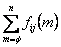and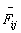( ) be the complementary probability distribution of Tij.(n)  = 1 - Fij (n) = P(Tij > n)  =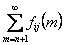Suppose the patient enters state i. Let Yi be the time he spent in state i before moving out of the state i. Then Yi is called the waiting time in state i.

We let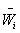(n) be the probability distribution function of Yi. Then

 wi (m)  =  P(Yi  =  m)  =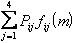The probability distribution Wi ( ) and the complementary cumulative probability distribution Wi ( ) for the waiting times are given as follows

 Wi (m) =  P(Yi  £  n)  =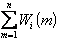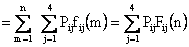and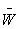i (n)   =  P(Yi  >  n)  =  1  -  Wi (n) = =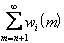=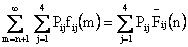(2)

Interval Transition Probabilities

We define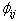(n) to be the probability that the leprosy patient will be in state j in time n given that he entered state i in time zero. This is called the interval transition probability from state i to state j in the interval (0, n]. Theni, j = 1, 2, 3, 4,            n = 1, , 3, . . . . (3)(n) is as defined in (2) .

The Weibull Distribution

The holding time in the states is described by the Weibull probability distribution function. The probability mass function of a discrete random variable T having the three parameter Weibull distribution is given byt < 0, a, b, c > 0

The scale parameter is α, the parameter β determines the shape and it is therefore called the shape parameter, the location parameter is c.

We can reduce the distribution to a two parameter Weibull by putting t1 = t - c, thus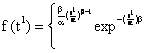a, b > 0

The parameters of the distribution have been estimated using percentile points presented in .

Model Assumptions

It is assumed that the transition from one state to a different state should not occur at time t = 0 (year 0) and that the basic unit of time is one year. A leprosy patient that dies during treatment or during the period of a follow-up is assumed to have died of leprosy. Natural death is not considered. A patient that fails to recover from leprosy after completing a dose of the treatment is considered to have developed a resistance to the drug and is consequently considered to have relapsed.

We consider a leprosy patient. Let us assume that each year the leprosy patient is under treatment or has recovered from the disease or has relapsed or has died from the disease. We therefore have a four state process State 1- Under treatment, State 2- Recovery, State 3- Relapse and State 4- Death due to leprosy. The transition from one state to another is indicated in the transition diagram below.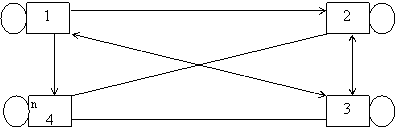Figure 1. Transition diagram for Leprosy Process

We observe that states 1, 2 and 3 are transient states and state 4 is an absorbing state. In other words, all possible transitions of the process are made between states 1, 2 and 3 but once a transition is made to state 4 the process terminates. We would like a transition to occur at a time the duration of stay in a state is completed, even if the new state is the same as the old. Such a transition is called a virtual transition, and is represented by loops in the transition diagram.

From the above transition diagram we can record the transition probability matrix ‘P’ for the process as shown below.Figure 2 shows a realization of the process.Figure 2. A possible realization of the process

The Effect of Treatment

The effect is measured by the increase in probability of recovery, a decrease in the probabilities of death and having a relapse. This is obtained from the following expressions.

 E12  =  (1 + k)P12 E1j  =  (1 - k)P1j,  j = 3, (4)

where k is a positive real number in the interval [0, 1). Then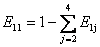and the transition matrix is P with the first row replaced by E1j.  i = 1, 2, 3, 4.

Application

A 10- year follow-up of a 6-week quadruple drug regimen for  136 multibacillary leprosy patients showed 13% relapses, seven late and the  remaining (111) were considered to have recovered from leprosy .

Result

Using equations (3) and (4), we obtain the results presented in Tables 2-4 and illustrated in figures 3-5 respectively.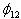(n) is the probability that a leprosy patient will recover at time n given that the patient started treatment at time zero, and that the treatment is k effective at zero percent, fifty percent and ninety-nine percent on the sensitivity analysis.

Table 2. The probabilities of recovery from leprosy -(n)

 n K = 0 K = 0.50 K = 0.99 1 0.0095754713 0.0143632062 0.0190551877 2 0.0220378209 0.0330567323 0.0438552648 3 0.0356860533 0.0535290837 0.0710152462 4 0.0496131815 0.0744197667 0.0987302288 5 0.0632850900 0.0949276388 0.1259373277 6 0.0763817355 0.1145725995 0.1519996524 7 0.0887717359 0.1330755502 0.1765469015 8 0.1001924053 0.1502885967 0.1993828863 9 0.1107673943 0.1661510915 0.2204271257 10 0.1204402894 0.1806604415 0.2396761775 11 0.1292348504 0.1938522607 0.2571773529 12 0.1371911019 0.2057866454 0.2730102837
Table 3. The probabilities of a relapse from lepros -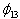(n)
 n K = 0 K = 0.50 K = 0.99 1 0.0063836472 0.0031918236 0.0000638364 2 0.0146918809 0.0073459405 0.0001469187 3 0.0237907022 0.0118953511 0.0002379068 4 0.0330554519 0.0165377259 0.0003307542 5 0.0421900600 0.0210950300 0.0004219002 6 0.0509211533 0.0254605766 0.0005092110 7 0.0591446869 0.0295723435 0.0005914463 8 0.0667949319 0.0333974659 0.0006679487 9 0.0738449320 0.0369224660 0.0007384486 10 0.0802935287 0.0401467644 0.0008029345 11 0.0861565620 0.0430782810 0.0008615648 12 0.0914607272 0.0457303636 0.0009146064

Table 4. The probabilities of death from lepros -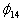(n)
 n K = 0 K = 0.50 K = 0.99 1 0.0031918236 0.0015959118 0.0000319182 2 0.0073459405 0.0036729702 0.0000734593 3 0.0118953511 0.0059476756 0.0001189534 4 0.0165377259 0.0082688630 0.0001653771 5 0.0213950300 0.0105475150 0.0002109501 6 0.0254605766 0.0127302883 0.0002546055 7 0.0295723435 0.0147861717 0.0002957231 8 0.0333974659 0.0166987330 0.0003339743 9 0.0369224660 0.0184612330 0.0003692243 10 0.0401467644 0.0200733822 0.0004014672 11 0.0430782810 0.0215391405 0.0004307824 12 0.0457303636 0.0228651818 0.0004573032Figure 3. The graph of the probabilities of recovery from leprosyFigure 4. The graph of the probabilities of a relapse from leprosy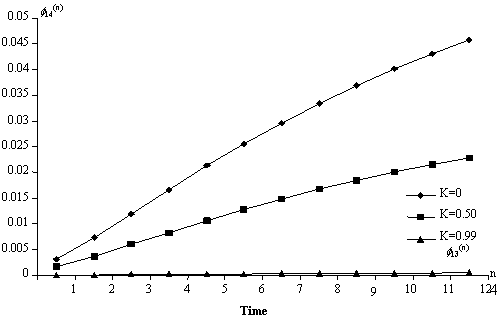Figure 5. The graph of the probabilities of death from leprosy

Discussion

The result presents the concept of control of leprosy such that cases of recovery are maximized and consequently minimizing the incidence of relapse and death due to leprosy. We observed from the tables and the graphs that when the treatment is 99% effective (k = 0.99), the probabilities of relapse and death from leprosy has been reduced to zero and consequently increased the probability of recovery to unity.

Semi -Markov model could be used as a predictive device to study leprosy conditions. Such predictions could be useful to the government and non-governmental organizations for the management of resources for the control of leprosy disease.

References

. Lechat M. F., The torments and blessings of the Leprosy epidemometric model, Leprosy Review, 1981, 52, p. 187-196.

. Bechelli L. M., Barrai I., Gallego P., Correlation between leprosy rates in villages different distances apart, Bulletin World Health Organization, 1973, 48, p. 257-378.

. Abubakar U. Y., A Study of Relapses in Leprosy: A Semi-Markov Approach, Abacus, The Journal of the Mathematical Association of Nigeria, 2004, 31(2B), p. 370-377.

. Ross S. M., Introduction to Probability Models, Academic Press, Inc.New York, 1989.

. Korve K. N., Models for the Management of asthma, PhD. Thesis, Faculty of Mathematical Studies, University of Southampton England, 1993.

. Howard R. A., Dynamic Probabilistic Systems, Vol.1&2, John Wiley, New York, 1971.

. Dubey, Some Percentile Estimators for Weibull Parameters, Technometrics, 1967, 9, p. 119-129.

. Pattyn S., Grillone S., Relapse rates and a 10-year follow-up of a 6-week quadruple drug regimen for multibacillary leprosy, Leprosy Review, 2002, 73, p. 245-247.